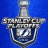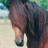# Fractal Boxes by Mobius for ThinkorSwim#### markos

##### Well-known member
VIPCode:
``````# Fractal Boxes
# Mobius 2017
# Altered Fractals to show Box Breakouts

input sequenceCount = 4;

def maxSideLength = sequenceCount + 10;
def upRightSide = fold i1 = 1 to maxSideLength + 1
with count1
while count1 != sequenceCount and
count1 != -1
do if GetValue(high, -i1) > high or
(GetValue(high, -i1) == high and
count1 == 0)
then -1
else if GetValue(high, -i1) < high
then count1 + 1
else count1;
def upLeftSide = fold i2 = 1 to maxSideLength + 1
with count2
while count2 != sequenceCount and
count2 != -1
do if GetValue(high, i2) > high or
(GetValue(high, i2) == high and
count2 >= 1)
then -1
else if GetValue(high, i2) < high
then count2 + 1
else count2;
def downRightSide = fold i3 = 1 to maxSideLength + 1
with count3
while count3 != sequenceCount and
count3 != -1
do if GetValue(low, -i3) < low or
(GetValue(low, -i3) == low and
count3 == 0)
then -1
else if GetValue(high, -i3) > low
then count3 + 1
else count3;
def downLeftSide = fold i4 = 1 to maxSideLength + 1
with count4
while count4 != sequenceCount and
count4 != -1
do if GetValue(low, i4) < low or
(GetValue(low, i4) == low and
count4 >= 1)
then -1
else if GetValue(low, i4) > low
then count4 + 1
else count4;

def UF = if upRightSide == sequenceCount and
upLeftSide == sequenceCount
then high
else UF;
def pUF = if UF != UF
then UF
else pUF;
def DF = if downRightSide == sequenceCount and
downLeftSide == sequenceCount
then low
else DF;
def pDF = if DF != DF
then DF
else pDF;
plot UpFractal = UF;
plot DownFractal = DF;
def trend = if UF > pUF and DF > pDF
then 1
else if UF < pUF and DF < pDF
then -1
else 0;
UpFractal.SetPaintingStrategy(PaintingStrategy.Horizontal);
UpFractal.AssignValueColor(if Trend == 1
then color.green
else if Trend == -1
then color.red
else color.white);
UpFractal.SetLineWeight(2);
DownFractal.SetPaintingStrategy(PaintingStrategy.Horizontal);
DownFractal.AssignValueColor(if Trend == 1
then color.green
else if Trend == -1
then color.red
else color.white);
DownFractal.SetLineWeight(2);
AddCloud(if Trend ==  1 then UpFractal else double.nan, DownFractal, Color.green, Color.green);
AddCloud(if Trend == -1 then UpFractal else double.nan, DownFractal, Color.red, Color.red);
AddCloud(if Trend ==  0 then UpFractal else double.nan, DownFractal, Color.white, Color.white);``````

Code Share: https://tos.mx/SEidEM

Last edited by a moderator:#### markos

##### Well-known member
VIP
This Fractal Boxes study may have alerted you to the downside break if it had alarms put on it.
Or the break beyond the Expected Move, possibly. Had to kill the premium sellers without a net (hedge) today.
It's all there in the chart share.
Could someone please backtest it on a few short timeframes?#### mc01439

##### Well-known member
2019 Donor
VIP
/CL (1) contract - raw signal to raw signal.

def sellsignal = Trend == -1 and close < DownFractal;
def buysignal = Trend == 1 and close > UpFractal;

AddOrder(OrderType.SELL_AUTO, sellsignal equals 1, close, 1, Color.RED, Color.RED);

Example:
5M-180days = +\$4,670 / 254 trades
10M-180days = -3,860 / 159 trades
15M-180days = -670 / 99 trades
30M-180days = +15,180.00 / 53 trades
1H-180days = +3,850.00 / 23 trades
4H-180days = -11,930 / 12 trades
1D-5years = +22,780 / 7 trades

•#### markos

##### Well-known member
VIP
Thanks @mc01439 !I guess it more of a consolidation zone to help keep nervous folks in a trade by showing them there's no need to panic until it breaks out. Any thoughts? Just curious on your take. Hope you had a good day, markos

Last edited:
T

#### Talochka

##### Member
Thanks @mc01439 !I guess it more of a consolidation zone to help keep nervous folks in a trade by showing them there's no need to panic until it breaks out. Any thoughts? Just curious on your take. Hope you had a good day, markos
Hello , where can I find Mobius_IMO_ms? Thanks

T

#### Talochka

##### Member
Thanks @mc01439 !I guess it more of a consolidation zone to help keep nervous folks in a trade by showing them there's no need to panic until it breaks out. Any thoughts? Just curious on your take. Hope you had a good day, markos
It is on the bottom of the screen#### markos

##### Well-known member
VIP
It is on the bottom of the screen
Have you been through the tutorials on this site yet? It's a pretty good place to start. The TMO oscillator by Mobius can be found in several places.
Here are two:
In this second entry you will find links to more TMO information. RSI Laguerre is closely associated with it, thus it is intertwined in many threads.

T

#### Talochka

##### Member
•markos
K

#### KarlWolff

##### Member
The boxes don't seem to refresh/generate automatically. Any idea why?#### markos

##### Well-known member
VIP
The boxes don't seem to refresh/generate automatically. Any idea why?
@KarlWolff there is nothing wrong with the study. It is just as Mobius created it. That being said, it completes the next fractal box when a box is complete according to your settings. Play with it under different time frames and fractal periods and you'll see how it might help you. Give it time and watch it work. If it doesn't suit you, that's ok.#### MattATM

##### Active member
VIP
Hello my friends this is what I am working on for the shorter time frames like 5 minutes...
What do you think about improving the accuracy???
Code:
``````# Fractal Boxes
# Mobius 2017
# Altered Fractals to show Box Breakouts
input MobiusFractalBoxTime = aggregationPeriod.thirtY_MIN;
def HIGHBOX = high(period = MobiusFractalBoxTime);
def LOWBOX = low(period = MobiusFractalBoxTime);

input sequenceCount = 40;

def maxSideLength = sequenceCount + 800;
def upRightSide = fold i1 = 1 to maxSideLength + 1
with count1
while count1 != sequenceCount and
count1 != -1
do if GetValue(high, -i1) > HIGHBOX or
(GetValue(high, -i1) == HIGHBOX and
count1 == 0)
then -1
else if GetValue(high, -i1) < HIGHBOX
then count1 + 1
else count1;
def upLeftSide = fold i2 = 1 to maxSideLength + 1
with count2
while count2 != sequenceCount and
count2 != -1
do if GetValue(high, i2) > HIGHBOX or
(GetValue(high, i2) == HIGHBOX and
count2 >= 1)
then -1
else if GetValue(high, i2) < HIGHBOX
then count2 + 1
else count2;
def downRightSide = fold i3 = 1 to maxSideLength + 1
with count3
while count3 != sequenceCount and
count3 != -1
do if GetValue(low, -i3) < LOWBOX or
(GetValue(low, -i3) == LOWBOX and
count3 == 0)
then -1
else if GetValue(high, -i3) > LOWBOX
then count3 + 1
else count3;
def downLeftSide = fold i4 = 1 to maxSideLength + 1
with count4
while count4 != sequenceCount and
count4 != -1
do if GetValue(low, i4) < LOWBOX or
(GetValue(low, i4) == LOWBOX and
count4 >= 1)
then -1
else if GetValue(low, i4) > LOWBOX
then count4 + 1
else count4;

def UF = if upRightSide == sequenceCount and
upLeftSide == sequenceCount
then high
else UF;
def pUF = if UF != UF
then UF
else pUF;
def DF = if downRightSide == sequenceCount and
downLeftSide == sequenceCount
then low
else DF;
def pDF = if DF != DF
then DF
else pDF;
plot UpFractal = UF;
plot DownFractal = DF;
def trend = if UF > pUF and DF > pDF
then 1
else if UF < pUF and DF < pDF
then -1
else 0;
UpFractal.SetPaintingStrategy(PaintingStrategy.Horizontal);
UpFractal.AssignValueColor(if Trend == 1
then color.green
else if Trend == -1
then color.red
else color.white);
UpFractal.SetLineWeight(2);
DownFractal.SetPaintingStrategy(PaintingStrategy.Horizontal);
DownFractal.AssignValueColor(if Trend == 1
then color.green
else if Trend == -1
then color.red
else color.white);
DownFractal.SetLineWeight(2);
AddCloud(if Trend ==  1 then UpFractal else double.nan, DownFractal, Color.green, Color.green);
AddCloud(if Trend == -1 then UpFractal else double.nan, DownFractal, Color.red, Color.red);
AddCloud(if Trend ==  0 then UpFractal else double.nan, DownFractal, Color.white, Color.white);``````

•A

##### Member
This works pretty nicely as both a range and trend indicator, one question however:
Does this Update in real time?The cloud appears to have a delay of over 0 bars, so would that mean I would not be able to catch the first run down (in the picture) until after four bars, which in this case might be fine but might take away potential profits?

Thanks for the study!

Last edited:Fractal Pivot Strategy Mobile by Mobius Questions 11Deviation Scaled VWAP with Fractal Energy for ThinkorSwim Indicators 42